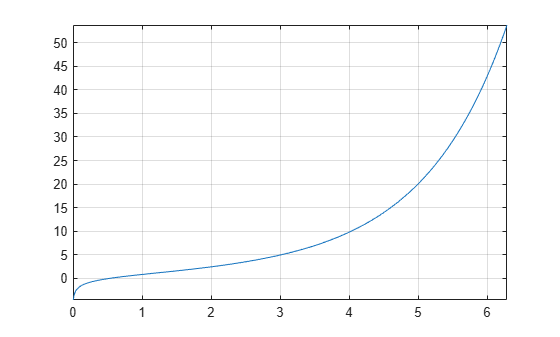# coshint

Hyperbolic cosine integral function

## Syntax

``coshint(X)``

## Description

example

````coshint(X)` returns the hyperbolic cosine integral function of `X`.```

## Examples

### Hyperbolic Cosine Integral Function for Numeric and Symbolic Arguments

Depending on its arguments, `coshint` returns floating-point or exact symbolic results.

Compute the hyperbolic cosine integral function for these numbers. Because these numbers are not symbolic objects, `coshint` returns floating-point results.

`A = coshint([-1, 0, 1/2, 1, pi/2, pi])`
```A = 0.8379 + 3.1416i -Inf + 0.0000i -0.0528 + 0.0000i 0.8379... + 0.0000i 1.7127 + 0.0000i 5.4587 + 0.0000i```

Compute the hyperbolic cosine integral function for the numbers converted to symbolic objects. For many symbolic (exact) numbers, `coshint` returns unresolved symbolic calls.

`symA = coshint(sym([-1, 0, 1/2, 1, pi/2, pi]))`
```symA = [ coshint(1) + pi*1i, -Inf, coshint(1/2), coshint(1), coshint(pi/2), coshint(pi)]```

Use `vpa` to approximate symbolic results with floating-point numbers:

`vpa(symA)`
```ans = [ 0.83786694098020824089467857943576... + 3.1415926535897932384626433832795i,... -Inf,... -0.052776844956493615913136063326141,... 0.83786694098020824089467857943576,... 1.7126607364844281079951569897796,... 5.4587340442160681980014878977798]```

### Plot Hyperbolic Cosine Integral Function

Plot the hyperbolic cosine integral function on the interval from 0 to `2*pi`.

```syms x fplot(coshint(x),[0 2*pi]) grid on```### Handle Expressions Containing Hyperbolic Cosine Integral Function

Many functions, such as `diff` and `int`, can handle expressions containing `coshint`.

Find the first and second derivatives of the hyperbolic cosine integral function:

```syms x diff(coshint(x), x) diff(coshint(x), x, x)```
```ans = cosh(x)/x ans = sinh(x)/x - cosh(x)/x^2```

Find the indefinite integral of the hyperbolic cosine integral function:

`int(coshint(x), x)`
```ans = x*coshint(x) - sinh(x)```

## Input Arguments

collapse all

Input, specified as a symbolic number, variable, expression, or function, or as a vector or matrix of symbolic numbers, variables, expressions, or functions.

collapse all

### Hyperbolic Cosine Integral Function

The hyperbolic cosine integral function is defined as follows:

`$\text{Chi}\left(x\right)=\gamma +\mathrm{log}\left(x\right)+\underset{0}{\overset{x}{\int }}\frac{\mathrm{cosh}\left(t\right)-1}{t}\text{\hspace{0.17em}}dt$`

Here, γ is the Euler-Mascheroni constant:

`$\gamma =\underset{n\to \infty }{\mathrm{lim}}\left(\left(\sum _{k=1}^{n}\frac{1}{k}\right)-\mathrm{ln}\left(n\right)\right)$`

 Cautschi, W. and W. F. Cahill. “Exponential Integral and Related Functions.” Handbook of Mathematical Functions with Formulas, Graphs, and Mathematical Tables. (M. Abramowitz and I. A. Stegun, eds.). New York: Dover, 1972.

#### Mathematical Modeling with Symbolic Math Toolbox

Get examples and videos